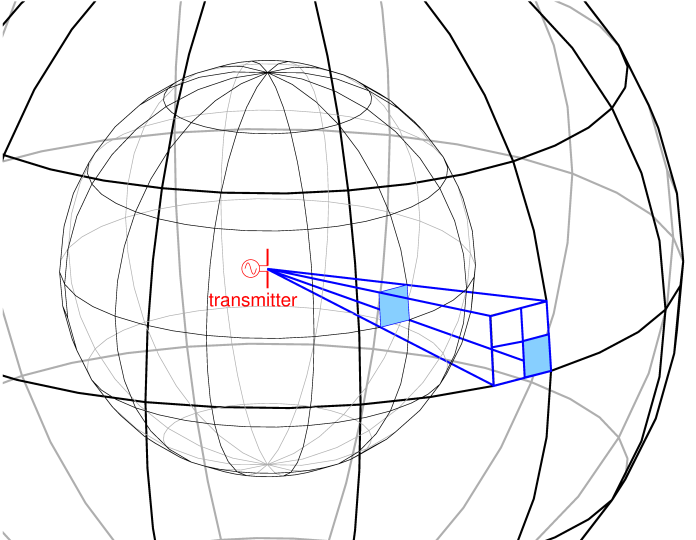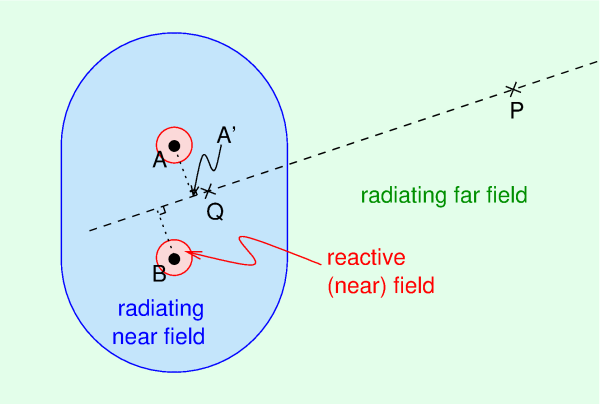# Near and far fields

Pieter-Tjerk de Boer, PA3FWM pa3fwm@amsat.org

(This is an adapted version of an article I wrote for the Dutch amateur radio magazine Electron, March 2021.)

Suppose we put somewhere in space, far away from obstacles like planets, a 100 watt transmitter with an isotropic antenna, i.e., an antenna that radiates equally much in all directions. Now measure the electric field strength (in volts per meter) and the magnetic field strength (in ampere per meter) at distances of 100 and 200 meters from the antenna. Of course, we expect the field strengths to be lower at 200 m distance, but by how much? As it turns out, we can easily reason about this.If one multiplies the electric field strength (in V/m) and the magnetic field strength (in A/m), one gets something of the form volts times amperes per square meter, i.e., watts / m². That is no coincidence: the outcome of that multiplication indicates how much power (watts) "flows" through a square meter.

The figure shows the transmitter and two imaginary spheres around it, with radiuses of 100 m and 200 m. In the surface of each sphere a blue square is indicated of (say) 1 by 1 meter. We measure the field strenghts at that square, and their product tells us how much power flows through the square.

The area of the small sphere is 4 × π × 1002, or about 120000 m2, so from the 100 watts we started with, 100/120000 = 0.8 mW pass through this square meter. The large sphere's radius is twice as large, so its surface area is 4 times as large. The same 100 watts are now spread out over a 4 times larger area. So the power per m2 is 4 times smaller. Indeed, in the figure we see that the blue square in the large sphere only intercepts a quarter of the radiation passing through the (equally large) blue square in the small sphere.

Now, if the power per m² has been reduced to a quarter, the electric and magnetic field strengths must each have been halved. (Their ratio is constant, 377 ohms in air or vacuum.) Thus, we conclude that the electric and magnetic field strength in a radiowave decrease like 1/r, where r is the distance to the source (transmit antenna): twice the distance, half the field strength.

That is, if the field is part of a radiowave.

## Loose magnets and loose charges

Everyone has at some time played with a magnet and a piece of metal attracted by that magnet. The farther away from that magnet, the weaker the attraction is. Does this also go as 1/r? I.e., twice as far, half the force? No, one easily feels that the attraction decreases much more quickly. In other words, not all fields decay as 1/r. (Actually this argument isn't quite right, because it's the magnet itself which magnetizes the metal; but that's not so important right now.)

Physicists have found out that the electric field of an electric charge decays as 1/r², so as the distance doubles, the field becomes four times weaker. And the magnetic field of a current in a wire also decays as 1/r².

If we take two equally large but opposite charges and put them at some distance from each other, like in a dipole antenna, each has a field decaying as 1/r². But farther away, the these fields increasingly cancel each other, because both charges are equally large but opposite. It turns out that the net remaining effect decreases as 1/r³.

## The near field and the far field

Now let's return to the dipole antenna from the first part. We see there's a current flowing in it, which, as we've just learned, produces a magnetic field decaying as 1/r². Furtermore we have equally large but opposite charges at the ends of the dipole, producing a net field that decays as 1/r³. That's worrying, since we expect our radiowave to have fields decaying as 1/r. These fields decay far too quickly. How's that possible?

The crucial point is that in the above, we only considered the fields caused by constant charges and constant currents: those produce constant electric and magnetic fields. But in an antenna the currents and charges are changing all the time, because the transmitter produces an alternating current. As a consequence, the fields are changing all the time too. And according to the laws of nature (more precisely, those laws known as Maxwell's laws) a varying electric field produces a magnetic field, and similarly, a varying magnectic field produces an electric field. The fields generated this way are again varying, so they againg produce new fields, and so on. With a bunch of mathematics, it turns out that this "chain reaction" leads to something which decays only as 1/r. And that is what we call radio waves or radiation.

The field near the dipole can be seen as the sum of two kinds of fields. On the one hand the fields produced directly by the charges and currents in the dipole, decaying as 1/r² or 1/r³; these fields dominate near the antenna, at small r, and are therefore called the "near" field. And on the other hand the fields belonging to the electromagnetic wave; these decay slower, namely as 1/r, so they dominate when we're farther away from the antenna, and are therefore called the "far" field.

## The reactive and the radiating field

A different name for the near field is the "reactive" field. Reactive is a posh word for what happens in capacitors and inductors. When one connects a capacitor to an AC source, it will be alternatingly charged and discharged: energy flows into the capacitor, is stored there as an electric field between the plates, and later delivered back to the source. An inductor does something similar, but stores the energy in a magnetic field.

The same happens in a (dipole) antenna: the electric and magnetic fields near the antenna are repeatedly created and removed. In other words, the antenna behaves as a capacitor and as an inductor. The fact that the energy is stored and returned within a period of the alternating current, immediately tells us that we must be talking about the field near the antenna. After all, nothing can travel faster than light, so if this "reactive" field would be too far away from the antenna, its energy could not be returned "in time".

Only a part of the energy is really radiated, so ends up in the part of the field that really leaves the antenna and decreases as 1/r: that part of the field is therefore also called the "radiating" field.

What happens to the energy in the "reactive" field? It can stay there, going back and forth between the electric and magnetic fields, like in case of a resonating LC circuit. A (usually small) part may also be converted into heat, in the resistance of the conductors and in dielectric losses of isolation material (including e.g. trees in the near field). The law of conservation of energy still remains valid: the transmitter delivers as much power to the antenna, as is radiated plus is lost in heat.

There is of course no strict border between the near and far fields, but often a distance of λ/2π is seen as the boundary: about 1/6 of the wavelength, so indeed near enough for returning to the dipole "in time".

## In between

If one has an antenna which is large compared to the wavelength, there is a third zone, kind of in between the other near and the far field. This is the "radiating near field". There we're already far enough from the antenna to have a really radiating field, so the energy is really leaving the antenna, but the radiation diagram doesn't yet have its final form.See the figure. We see two dipoles A and B, perpendicular to the screen, one wavelength away from each other, connected to one transmitter; or they could be two elements of a yagi antenna, for this story that doesn't matter. The field produced by both dipoles together is the sum of their individual fields, taking into account the phase difference between them; depending on the phase, the contributions of both dipoles can reenforce or cancel each other. Those phase differences are caused by the difference in the distances from the point where we measure to the two dipoles. If one is far away, e.g. at P, the distance difference can well be approximated by the measuring the distance along the dashed line: the difference between PA and PA' is very small. But as one gets closer, e.g. at Q, this isn't true anymore: QA is much longer than QA'. So if one is far away enough, our position on the dashed line doesn't matter for the distance difference: the radiation diagram then only depends on the direction, but not on how far away one is.

That is the important difference between the radiating near field and the (also radiating) far field: in the radiating near field the radiation diagram of the antenna isn't finalized yet. As a boundary between the two a distance of 2D²/λ is often assumed, where D is the size of the antenna.

As an (extreme) example, considers the Dwingeloo radio telescope's 25 m diameter dish, used at a wavelength of 23 cm. The reactive near field will already end at a distance of about λ/2π, that's about 4 cm. But the radiating near field goes on until about 2D²/λ, which is about 5 km! Practically this means that only some 5 km away from the dish the radiation diagram is a nicely shaped beam; closer to the dish there are still irregularities in it.